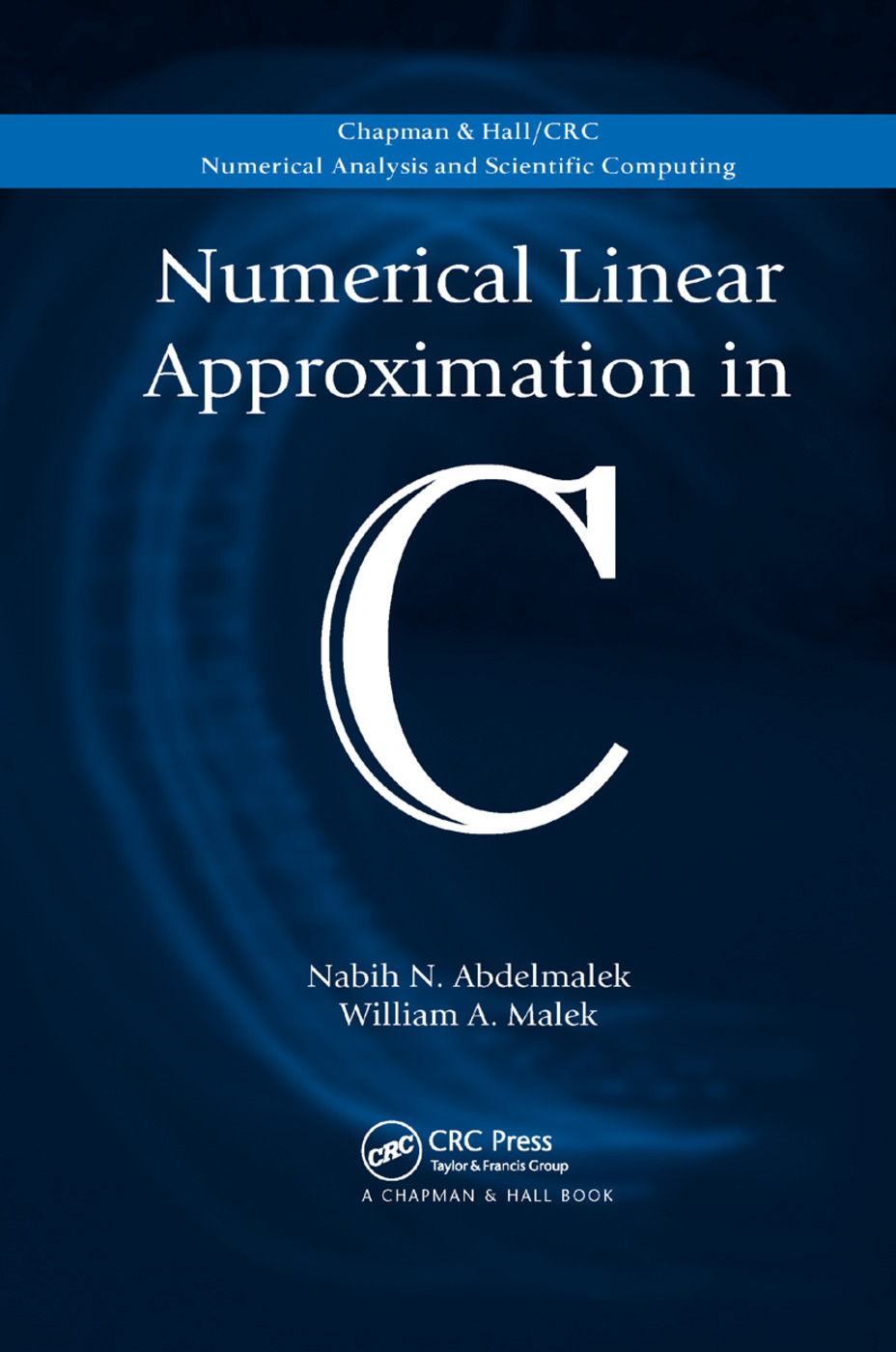Numerical Linear Approximation in C

1st Edition

Chapman and Hall/CRC

968 pages

Purchasing Options:\$ = USD
Paperback: 9780367387310
pub: 2019-12-20
SAVE ~\$14.99
Available for pre-order. Item will ship after 20th December 2019
\$74.95
\$59.96
x
Hardback: 9781584889786
pub: 2008-05-19
SAVE ~\$31.00
\$155.00
\$124.00
x
eBook (VitalSource) : 9780429144622
pub: 2008-05-19
from \$28.98

FREE Standard Shipping!

Description

Illustrating the relevance of linear approximation in a variety of fields, Numerical Linear Approximation in C presents a unique collection of linear approximation algorithms that can be used to analyze, model, and compress discrete data. Developed by the lead author, the algorithms have been successfully applied to several engineering projects at the National Research Council of Canada.

Basing most of the algorithms on linear programming techniques, the book begins with an introductory section that covers applications, the simplex method, and matrices. The next three parts focus on various L1, Chebyshev, and least squares approximations, including one-sided, bounded variables, and piecewise. The final section presents the solution of underdetermined systems of consistent linear equations that are subject to different constraints on the elements of the unknown solution vector.

Except in the preliminary section, all chapters include the C functions of the algorithms, along with drivers that contain numerous test case examples and results. The accompanying CD-ROM also provides the algorithms written in C code as well as the test drivers. To use the software, it is not required to understand the theory behind each function.

Applications of Linear Approximation. Preliminaries. Linear Programming and the Simplex Algorithm.Efficient Solutions of Linear Equations.Linear L1 Approximation.One-Sided L1 Approximation.L1 Approximation with Bounded Variables.L1 Polygonal Approximation of Plane Curves.Piecewise L1 Approximation of Plane Curves. Linear Chebyshev Approximation.One-Sided Chebyshev Approximation.Chebyshev Approximation with Bounded Variables.Restricted Chebyshev Approximation.Strict Chebyshev Approximation.Piecewise Chebyshev Approximation.Solution of Linear Inequalities.Least Squares and Pseudo-Inverses of Matrices.Piecewise Linear Least Squares Approximation.Solution of Ill-Posed Linear Systems.L1 Solution of Underdetermined Linear Equations.Bounded and L1 Bounded Solutions of Underdetermined Linear Equations.Chebyshev Solution of Underdetermined Linear Equations. Bounded Least Squares Solution of Underdetermined Linear Equations. Appendices. Index.